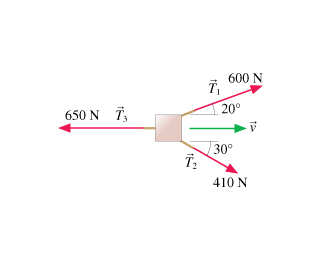# Problem: The three ropes shown in the birds-eye view of the figure are used to drag a crate 3.6 m across the floor. How much work is done by each of the three forces?

###### FREE Expert Solution
89% (173 ratings)
###### Problem Details
The three ropes shown in the birds-eye view of the figure are used to drag a crate 3.6 m across the floor. How much work is done by each of the three forces?Frequently Asked Questions

What scientific concept do you need to know in order to solve this problem?

Our tutors have indicated that to solve this problem you will need to apply the Intro to Calculating Work concept. You can view video lessons to learn Intro to Calculating Work. Or if you need more Intro to Calculating Work practice, you can also practice Intro to Calculating Work practice problems.

How long does this problem take to solve?

Our expert Physics tutor, Julia took 2 minutes and 4 seconds to solve this problem. You can follow their steps in the video explanation above.

What professor is this problem relevant for?

Based on our data, we think this problem is relevant for Professor Mccluskey's class at WSU.